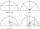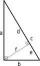Square

Rectangular square has side lengths 183 and 244 meters. How many meters will measure the path that leads straight diagonally from one corner to the other?

Result

x =  305 m

Solution:Leave us a comment of example and its solution (i.e. if it is still somewhat unclear...):Be the first to comment!To solve this example are needed these knowledge from mathematics:

Pythagorean theorem is the base for the right triangle calculator.

Next similar examples:

1. Reverse Pythagorean theoremGiven are lengths of the sides of the triangles. Decide which one is rectangular: Δ ABC: 77 dm, 85 dm, 36 dm ? Δ DEF: 55 dm, 82 dm, 61 dm ? Δ GHI: 24 mm, 25 mm, 7 mm ? Δ JKL: 32 dm, 51 dm, 82 dm ? Δ MNO: 51 dm, 45 dm, 24 dm ?
2. Height UTHow long is height in the equilateral triangle with a side b = 43?
3. Trio 2Decide whether trio of numbers is the side of a right triangle: 26,24,10.
4. Is right?Is triangle with sides 51, 56 and 77 right triangle?Ladder 10 meters long is staying against the wall so that its bottom edge is 6 meters away from the wall. What height reaches ladder?Ladder 6.4 meters long is positioned in the well such that its lower end is distanced from the wall of the well 1.2 m. The upper part of the ladder is supported on the upper edge of the well. How high is the well?8.3 meters long ladder is leaning against the wall of the well, and its lower end is 1.2 meters from this wall. How high from the bottom of a well is the top edge of the ladder?
8. HypotenuseCalculate the length of the hypotenuse of a right triangle with a catheti 71 cm and 49 cm long.Ladder 8 m long is leaning against the wall. Its foot is 1 m away from the wall. In which height ladder touches the wall?
10. BaseCompute base of an isosceles triangle, with the arm a=20 cm and a height above the base h=10 cm.
11. Numeric questionIt is possible negative irrational number?
12. Four ropesTV transmitter is anchored at a height of 44 meters by four ropes. Each rope is attached at a distance of 55 meters from the heel of the TV transmitter. Calculate how many meters of rope were used in the construction of the transmitter. At each attachment.
13. MedianIn the right triangle are sides a=41 dm b=42 dm. Calculate the length of the medians tc to the hypotenuse.
14. Broken treeThe tree is broken at 4 meters above the ground and the top of the tree touches the ground at a distance of 5 from the trunk. Calculate the original height of the tree.
15. WindbreakA tree at a height of 3 meters broke in the windbreak. Its peak fell 4.5 m from the tree. How tall was the tree?
16. The ditchDitch with cross section of an isosceles trapezoid with bases 2m 6m are deep 1.5m. How long is the slope of the ditch?
17. Chord circleThe circle to the (S, r = 8 cm) are different points A, B connected segment /AB/ = 12 cm. AB mark the middle of S'. Calculate |SS'|. Make the sketch.